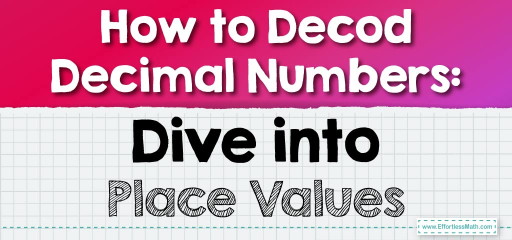# How to Decod Decimal Numbers: Dive into Place Values

Decimals are more than just dots in numbers; they help us represent fractions and parts of a whole. To truly understand decimals, it's essential to grasp the concept of place values. Just as with whole numbers, every position in a decimal number has a specific value. Let's explore this further!## Place Values within Decimal Numbers

### Example 1:

Decimal Number: $$2.345$$

To understand the place values:

– The digit $$2$$ is in the ones place.

– The digit $$3$$ is in the tenths place, representing $$3/10$$ or $$0.3$$.

– The digit $$4$$ is in the hundredths place, representing $$4/100$$ or $$0.04$$.

– The digit $$5$$ is in the thousandths place, representing $$5/1000$$ or $$0.005$$.

Starting from the left of the decimal point, the place values are ones, tenths, hundredths, thousandths, and so on. So, $$2.345$$ can be read as “two and three hundred forty-five thousandths.”

The Absolute Best Book for 5th Grade Students

### Example 2:

Decimal Number: $$7.89$$

To understand the place values:

– The digit $$7$$ is in the ones place.

– The digit $$8$$ is in the tenths place, representing $$8/10$$ or $$0.8$$.

– The digit $$9$$ is in the hundredths place, representing $$9/100$$ or $$0.09$$.

The number $$7.89$$ can be read as “seven and eighty-nine hundredths.”

Remember understanding the place values within decimal numbers is crucial for various mathematical operations, such as rounding, comparing, and performing arithmetic. With a solid grasp of place values, you’ll find working with decimals a breeze!

### Practice Questions:

1. Identify the digit in the tenths place: $$4.56$$

2. What is the value of the digit in the hundredths place in $$3.72$$?

3. Which digit is in the thousandths place in $$0.913$$?

4. What is the value of the digit in the tenths place in $$5.48$$?

5. Identify the digit in the hundredths place: $$6.034$$

A Perfect Book for Grade 5 Math Word Problems!

1. $$5$$

2. $$0.02$$

3. $$3$$

4. $$0.4$$

5. $$3$$

The Best Math Books for Elementary Students

### What people say about "How to Decod Decimal Numbers: Dive into Place Values - Effortless Math: We Help Students Learn to LOVE Mathematics"?

No one replied yet.

X
51% OFF

Limited time only!

Save Over 51%

SAVE $15 It was$29.99 now it is \$14.99